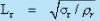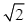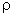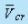Courses

# Exercise Problem - Principles of Physical Similarity and Dimensional Analysis Mechanical Engineering Notes | EduRev

## Mechanical Engineering : Exercise Problem - Principles of Physical Similarity and Dimensional Analysis Mechanical Engineering Notes | EduRev

The document Exercise Problem - Principles of Physical Similarity and Dimensional Analysis Mechanical Engineering Notes | EduRev is a part of Mechanical Engineering category.
All you need of Mechanical Engineering at this link: Mechanical Engineering

Exercise Problems  -  Chapter 6

1.  A 1/6 model automobile is tested in a wind tunnel with same air properties as the prototype. The prototype automobile runs on the roads at a velocity of 60 km/hr. For dynamically similar conditions, the drag measured on the model is 500 N. Determine the drag of the prototype and the power required to overcome this drag.

(500N, 8.33 KN)

2. A model is built of a flow phenomenon which is governed by the action of gravity and surface tension force. Show that the length scale ratio which will ensure complete similarity between model and the prototype is3. The speed of propagation U of a capillary wave in deep water is known to be a function of density ρ , wave length λ , and surface tension α . Using Dimensional Analysis, find out a relationship of U with ρ , λ , and α . (b) For a given surface tension and wavelength, how does the propagation speed changes if the density is halved ?

(increased by a factor of)

4.  A hydraulic jump occurring in a stilling basin is to be studied in a 1:36 scale model. The prototype jump has an initial velocity of 10 m/s, an entrance Froude number of 6.0 and a power loss of 2 kW per meter width of basin. Determine (a) the corresponding model velocity, (b) model Froude number and (c) power loss per meter width of the model

(a 1�67 m/s, (b) 6� 0, (c) 0�26 W)

5. A model of a reservoir having a free water surface within it is drained in 3 minutes by opening a sluice gate. The geometrical scale of the model is 1/100. How long would it take to empty the prototype?

(30 minutes)

6. Assuming that nothing is known about the particle motion under gravity beyond   x = G(ν0, g, t) where x, v0 , g, and t are respectively the displacement, initial velocity, gravitational acceleration, and time. Perform a dimensional analysis to explain the situation.

7. The tensile force inside a pendulum is known to depend on the mass, length, period, and angular amplitude of the pendulum. Perform a dimensional analysis.

8. The pressure drop in pipe flows of liquids is found to depend on the time required to pass a volume of a given liquid through, on this volume, and on the density as well as the viscosity of the liquid. Perform a dimensional analysis in a step by step manner, with the pressure drop and the density displayed as leading quantities.

9. Using the long steps of dimensional analysis, reduce the relationship n = G (gAM) for the frequency n of the wing beat of a flying insect, where g stands for the gravitational acceleration; A, the wing area;, the air density; and M, the mass of the insect. Choose and A as the leading quantities.

10. The shape of a drop of liquid pulsates as it falls. The period of oscillation is observed to depend on the surface tension, the mean radius of the drop, and the liquid density. Perform a dimensional analysis to express the period of oscillation..

11. Liquid flows across an orifice loses useful power which is dependent on the liquid density and viscosity, the volume flow rate, and the orifice diameter. Perform a dimensional analysis (with the objective of analysing power loss).

12. During the flow through a pipe, it is observed that there exists a critical average flow velocity, beyond which the flow becomes turbulent. It is also known thatis influenced by the diameter of the pipe, the density and the viscosity of the fluid. Perform a dimensional analysis to explain the situation.

In this course you have learnt the following

• Physical similarities are always sought between the problems of same physics. The complete physical similarity requires geometric similarity, kinematic similarity and dynamic similarity to exist simultaneously.
• In geometric similarity, the ratios of the corresponding geometrical dimensions between, the systems remain the same. In kinematic similarity, the ratios of corresponding motions and in dynamic similarity, the ratios of corresponding forces between the systems remain the same.
• For prediction of the performance characteristics of actual systems in practice from the results of model scale experiments in laboratories, complete physical similarity has to be achieved between the prototype and the model.
• Dimensional homogeneity of physical quantities implies that the number of dimensionless independent variables are smaller as compared to the number of their dimensional counterparts to describe a physical phenomenon. The dimensionless variables represent the criteria of similarity. Buckingham’s π theorem states that if a physical problem is described by m dimensional variables which can be expressed by n fundamental dimensions, then the number of independent dimensionless variables deﬁning the problem will be m-n. These dimensionless variables are known as π terms. The independent π terms of a physical problem are determined either by Buckingham’s π theorem or by Rayleigh’s indicial method
Offer running on EduRev: Apply code STAYHOME200 to get INR 200 off on our premium plan EduRev Infinity!

,

,

,

,

,

,

,

,

,

,

,

,

,

,

,

,

,

,

,

,

,

;